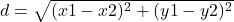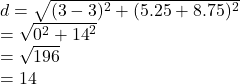## What is the distance between (3, 5.25) and (3, –8.75)?

Question

What is the distance between (3, 5.25) and (3, –8.75)?

in progress 0
1 month 2021-08-03T21:42:48+00:00 1 Answers 2 views 0

## 14 units

Step-by-step explanation:

The distance between two points can be found by using the formulawhere

(x1 , y1) and (x2 , y2) are the points

From the question the points are

(3, 5.25) and (3, –8.75)

The distance between them isWe have the final answer as

### 14 units

Hope this helps you